[[d.project_title]][[d.material_name]]# Mind+Python奥运射击

前段时间东京奥运会进行得如火如荼，奥运健儿们在赛场上奋力拼搏，给我们带来了精彩纷呈的比赛。其中，射击项目可谓激动人心。我国运动员杨倩就在该项目上夺得了奥运首金。联想到最近刚刚学习python上的pinpong库，突发奇想。觉得可以通过一个掌控板，模拟奥运会上奥运健儿们手中的射击武器，制作一个奥运射击小游戏。通过控制手中的掌控板，体验奥运健儿在射击赛场上的激动与不易。        致敬中国奥运运动员。无论如何，你们都是最棒的！

1. 创意方面：通过掌控板的水平仪功能控制电脑上的射击位置，射击时的靶子等图片由python上的turtle函数显示。水平仪控制射击区别于其他射击方式更为新颖，且更考验玩家的耐心与冷静。

2. 功能实现方面：玩家通过控制掌控板的倾斜程度进行对靶子的瞄准，通过按压掌控板上的按钮进行发射，射击效果与结果由turtle函数反映，同时掌控板上的屏幕也会反映相应数据。

1x

连接掌控板，在python的pinpong库中导入HPSensor（该函数可以读取掌控板返回的各种数据）。设置在循环中，由turtle函数显示的射击目标点移动到坐标为(x,y)的位置，x和y的值通过get_accelerometer（即读取掌控板的倾斜程度）得出。如此，玩家已可通过掌控板控制射击位置。

首先得导入两幅图，一幅是靶子，一幅是弹孔（得提前用画图工具控制好大小，且必须用gif格式）。将靶子与弹孔作为两支画笔的形状。靶子放在turtle显示的屏幕中心，使靶心与坐标（0,0）重合。弹孔暂时不显示。当掌控板A按钮被点击时，使弹孔移动到射击目标点的相应位置并显示（射击目标点一直由掌控板控制，同时弹孔应时刻保持抬笔）。如此，射击设置已基本完成。

使用math库中的hypot函数，求出弹孔（即射击到的位置）到坐标（0,0）（即靶心）的距离。根据靶子每环的宽度，定义函数，以求出的距离所在的各个范围返回各个值（该值即打中了的环数），通过turtle显示在电脑上。同时，计算累计分数与剩余枪数（共十枪），显示在掌控板的屏幕上。

游戏开始前显示规则，十枪打完即游戏结束，游戏结束时显示得分。可惜python中pinpong库，无法展现音效，使作品稍显不足。

```	                    					```import time,math,turtle
import numpy as np
from pinpong.board import Board,Pin
from pinpong.extension.handpy import HPSensor,HPScreen
Board("handpy").begin()
esp32 = HPScreen()
esp = HPSensor()
t0=turtle.Turtle()
tx=turtle.Turtle()
t1=turtle.Turtle()
t2=turtle.Turtle()
t2.pu()
t2.ht()
wn=turtle.Screen()
p1=np.array([0,0])
fa=r'C:\Users\ASUS\Desktop\a.gif'
fb=r'C:\Users\ASUS\Desktop\b.gif'
t0.shape(fa)
tx.shape('classic')
tx.left(90)
tx.goto(0,0)
tx.pu()
tx.color('red')
a=0
b=0
def j(p4):
if p4<20:
return 10
if 20<=p4<40:
return 9
if 40<=p4<60:
return 8
if 60<=p4<80:
return 7
if 80<=p4<100:
return 6
if 100<=p4<120:
return 5
if 120<=p4<140:
return 4
if 140<=p4<160:
return 3
if 160<=p4<180:
return 2
if 180<=p4<200:
return 1
if 200<=p4<220:
return 0
if 220<=p4<240:
return -1
if 240<=p4<260:
return -2
if p4>=260 :
return -5
esp32.display_in_line("游戏开始",1)
esp32.display_in_line("控制掌控板打靶",2)
esp32.display_in_line("靶子最外为-2环，以此",3)
esp32.display_in_line("类推。共有十颗子弹",4)
time.sleep(3)
esp32.fill_screen("black")
while True:
esp32.display_in_line('得分:{}'.format(a),1)
esp32.display_in_line('剩余子弹:{}'.format(10-b),2)
tx.shape('classic')
y=esp.get_accelerometer_Y()
x=esp.get_accelerometer_X()
tx.goto(-y,-x)
if esp.buttonA_is_pressed()==True :
t1.pu()
t1.goto(-y,-x)
t1.shape(fb)
p2=np.array([-y,-x])
p3=p2-p1
px=math.hypot(p3,p3)
t2.goto(300,200-b*10)
c=j(px)
a+=c
esp32.display_in_line('+{}'.format(c),3)
b+=1
if c>-3:
t2.write("第{}枪：{}环".format(b,c),False,'center',font=('华文中宋',9,'normal'))
if c<-3:
t2.write("第{}枪：打飞".format(b),False,'center',font=('华文中宋',9,'normal'))
time.sleep(0.3)
if b==10:
esp32.display_in_line('剩余子弹:{}'.format(10-b),2)
esp32.display_in_line('游戏结束，得分:{}'.format(a),1)
esp32.display_in_line('',3)
break
wn.exitonclick()    ```
```

通过前面几个步骤的制作，一款水平仪射击小游戏就完成了。作为一名学生，计算机中的“小白”，在作品制作的过程中，我有两点体会： 1. 作品要追求完整性。麻雀虽小五脏俱全，各种细节应精心琢磨，尝试，设置，才能使作品更上一层楼。 2. 好的学生作品离不开老师的精心指导。

在制作过程中，在我们的”地下铁”老师无微不至的指导帮助下，我们携手克服了一个又一个的技术难题，最终完成了这部作品。在这里，我想对老师说：“老师，您辛苦了！”

最后是作品完成后的一些图片和介绍视频。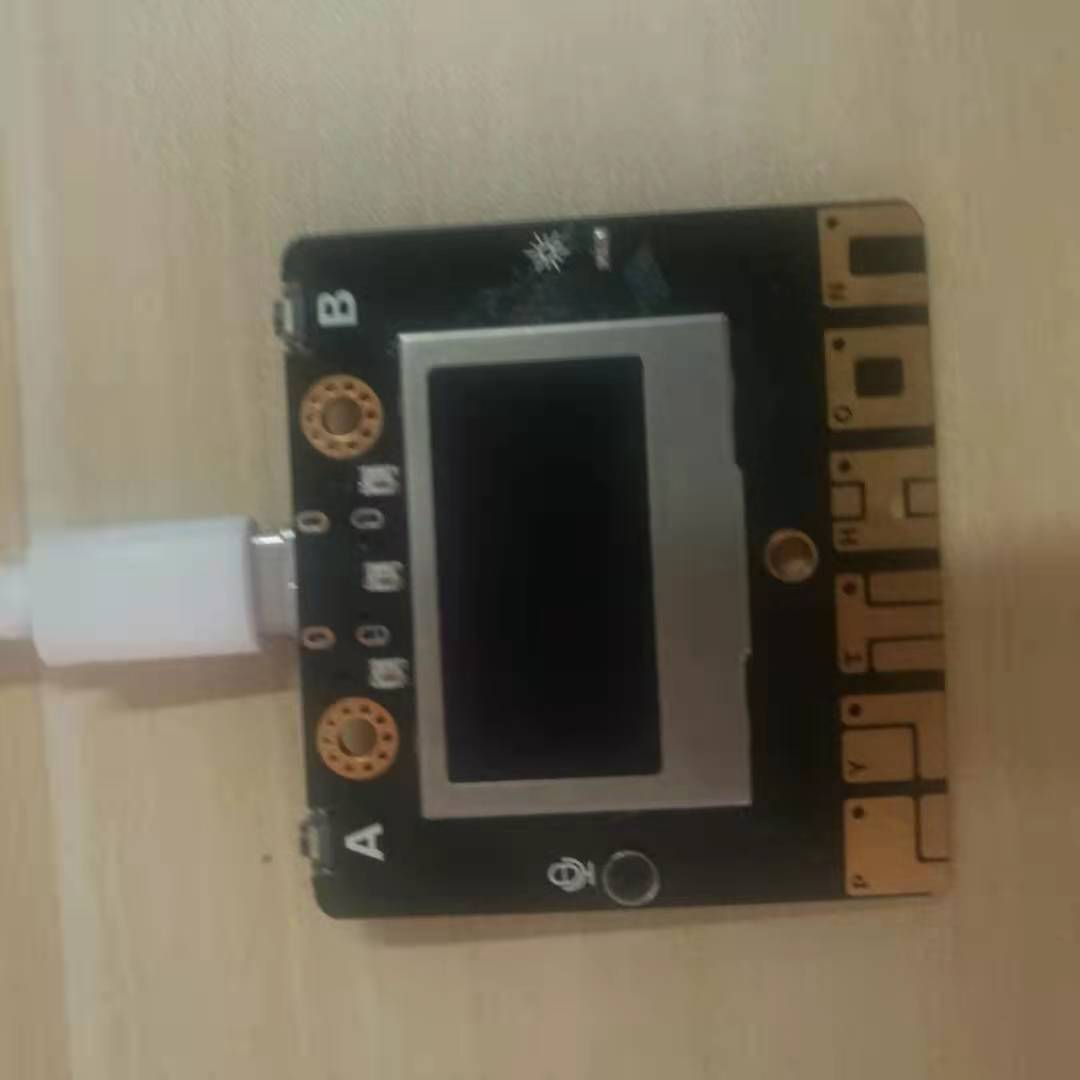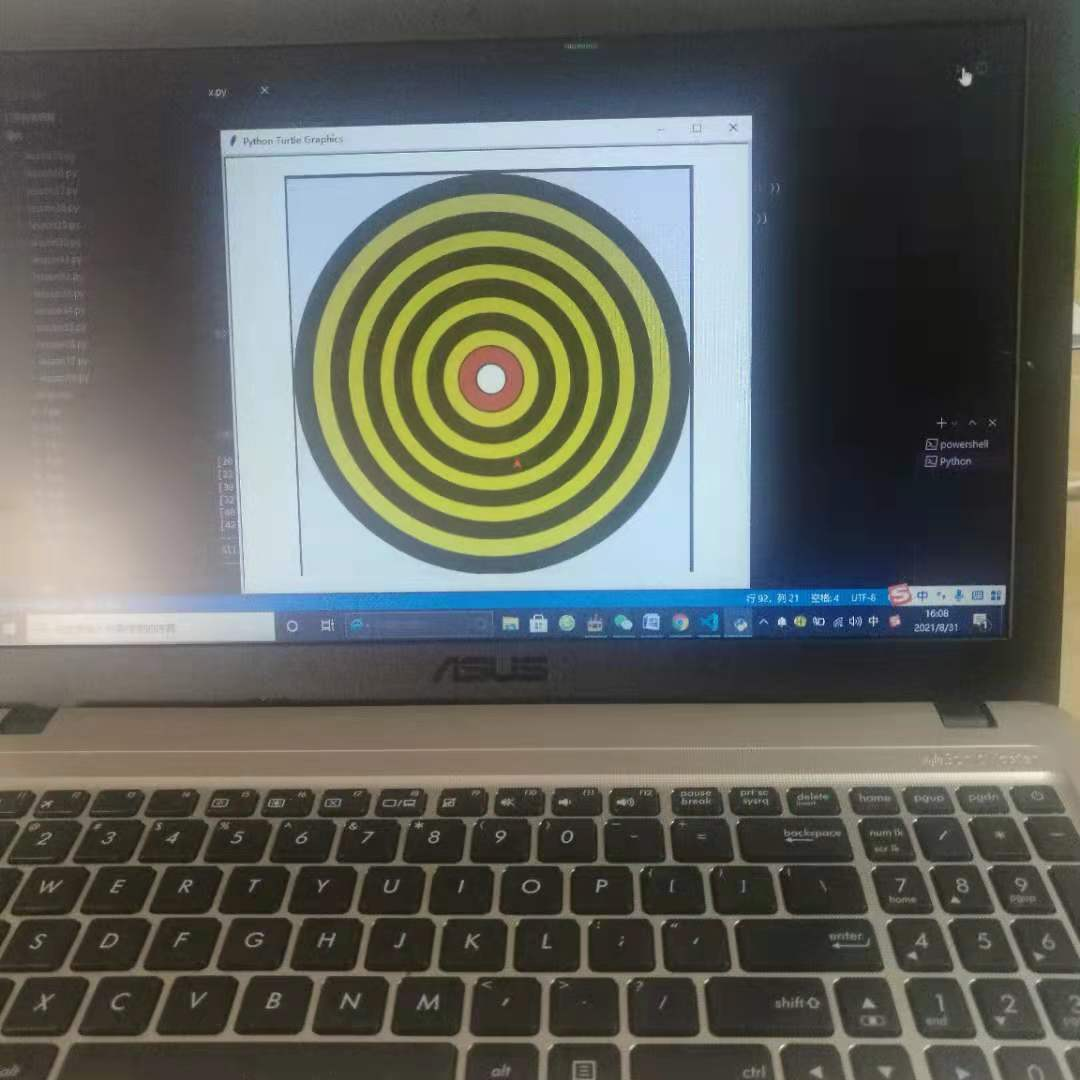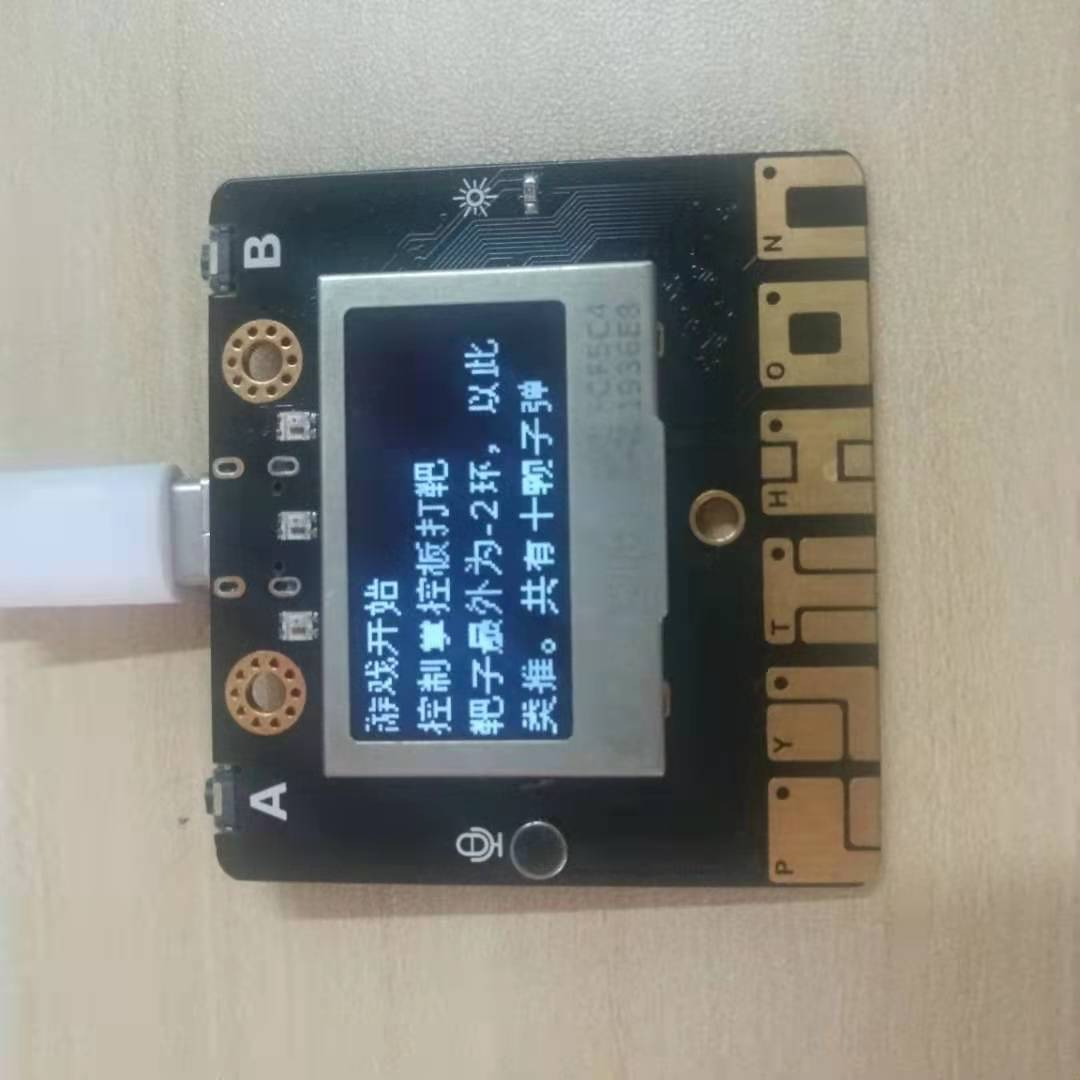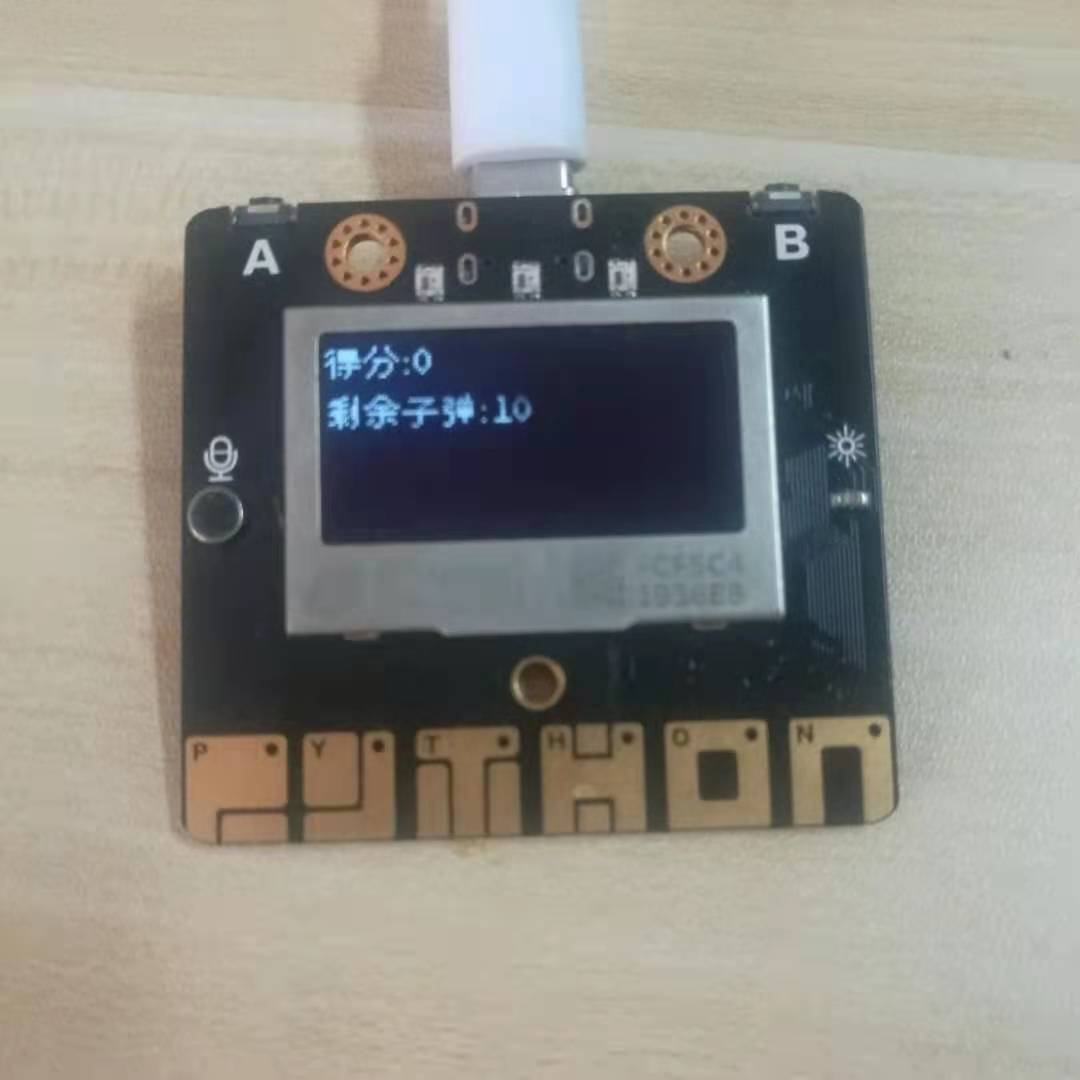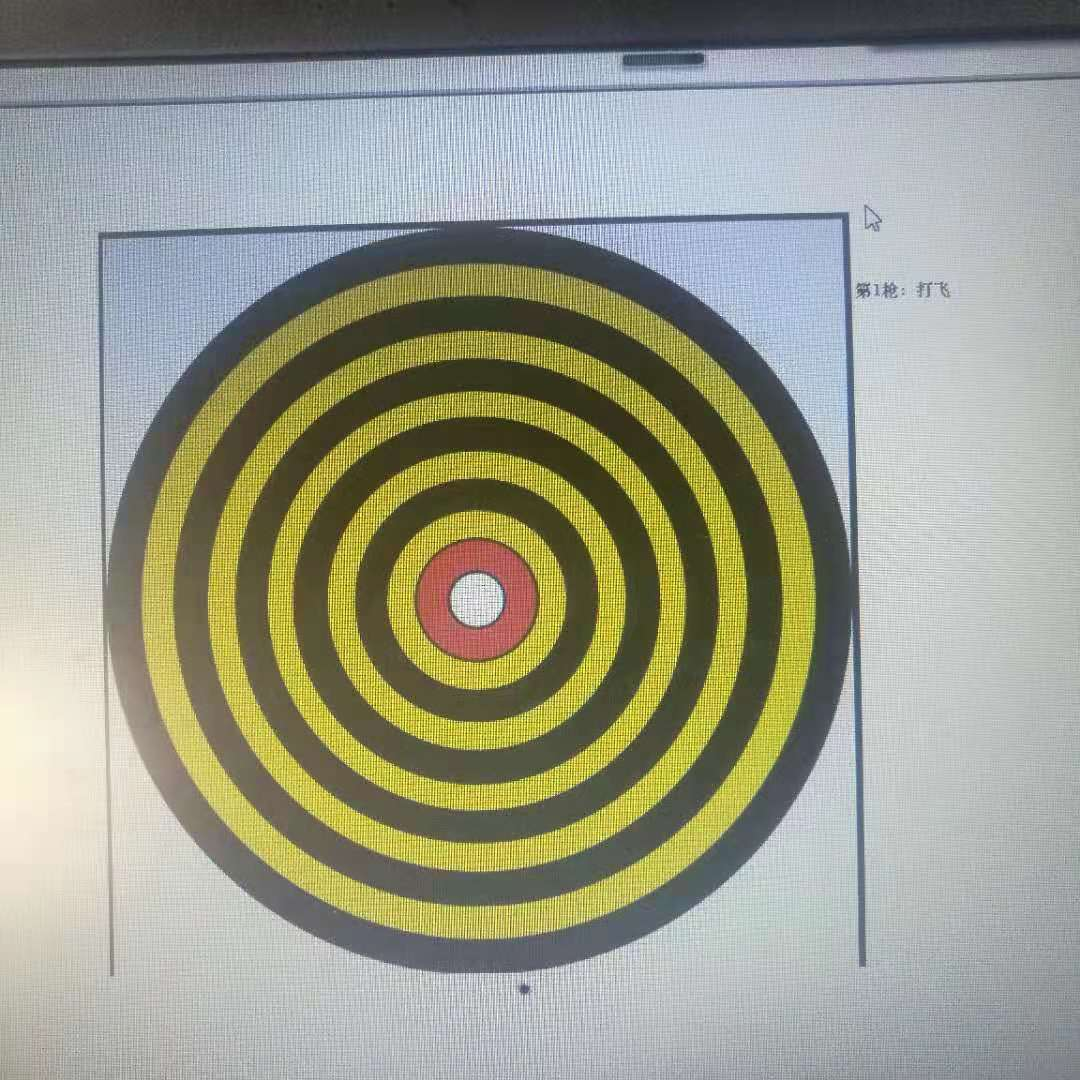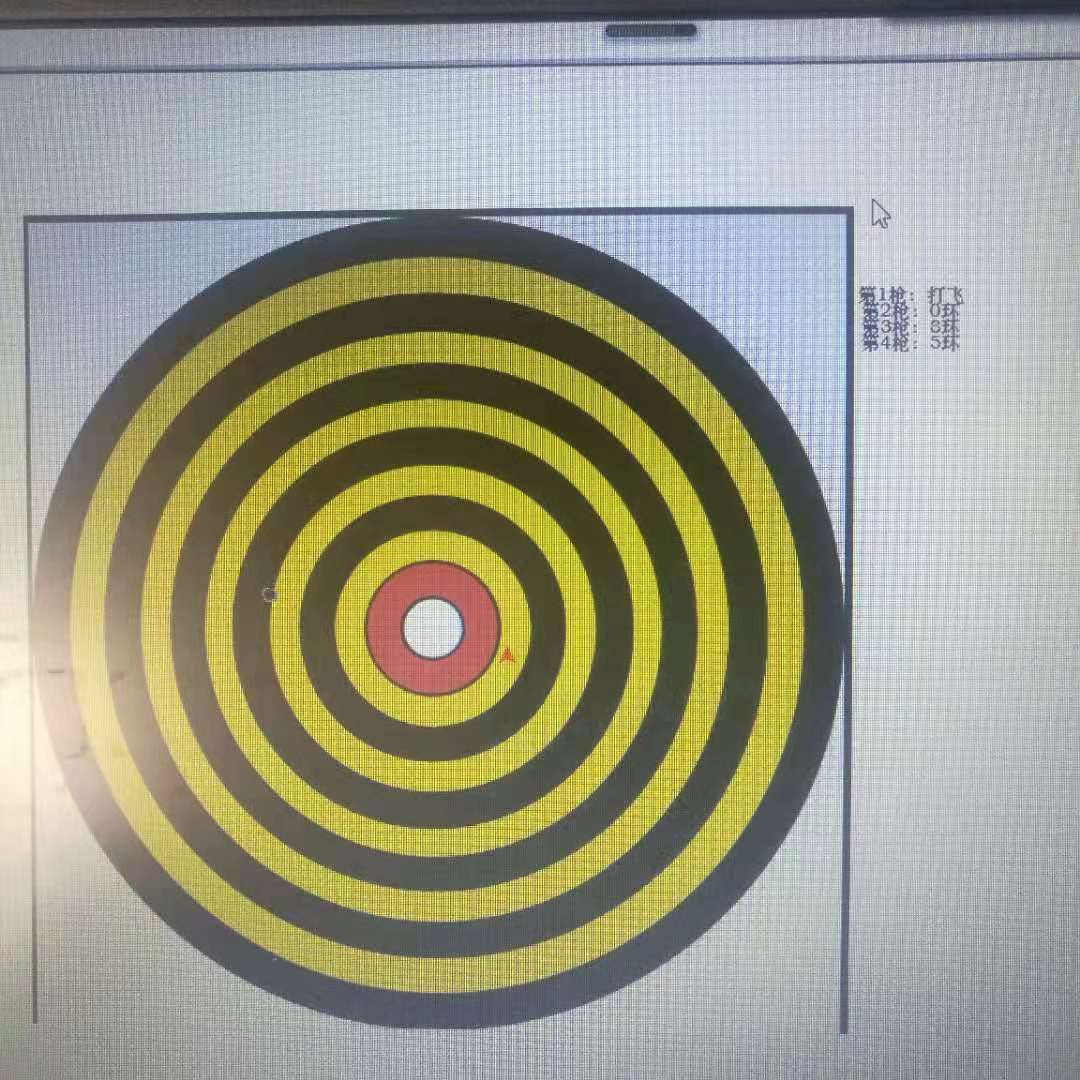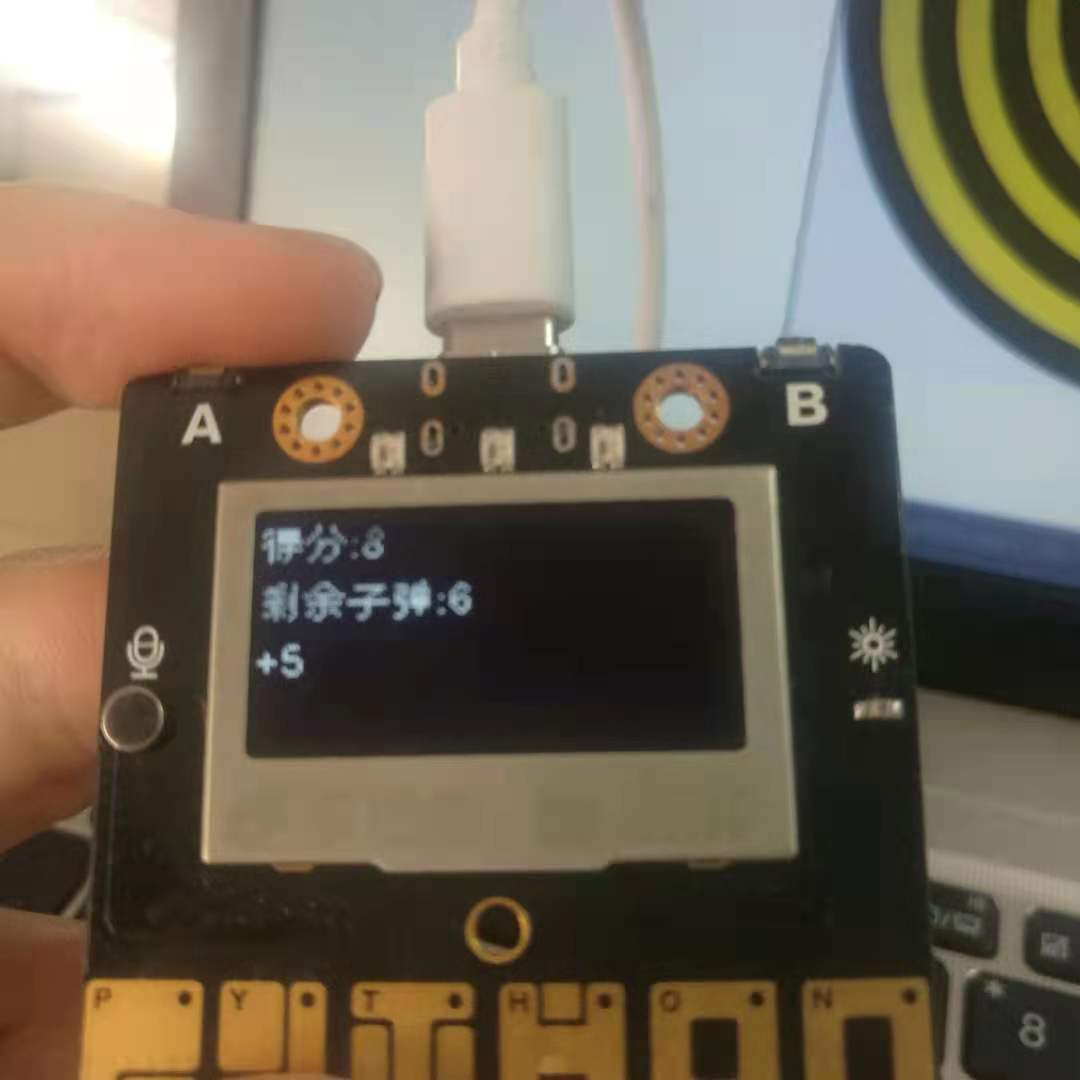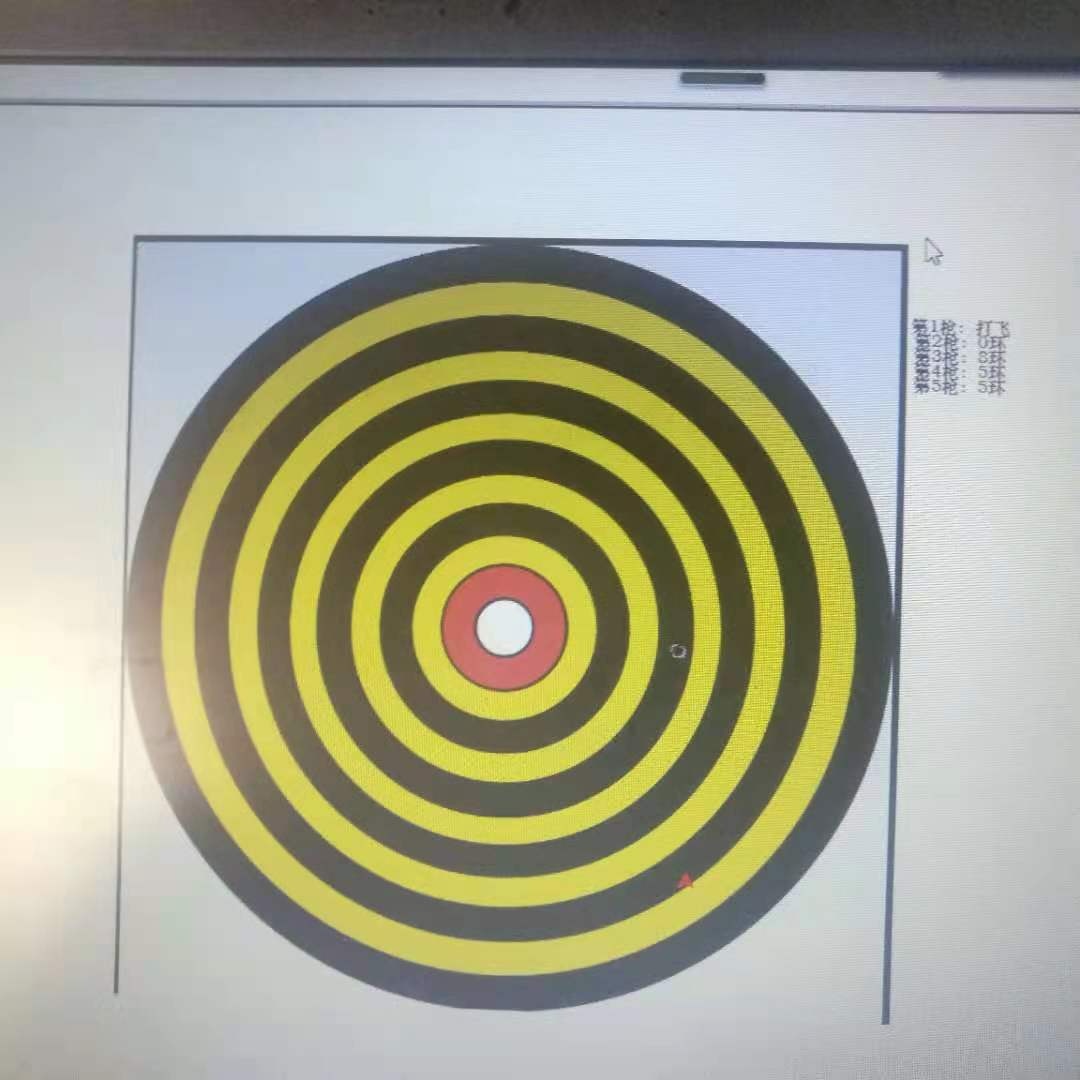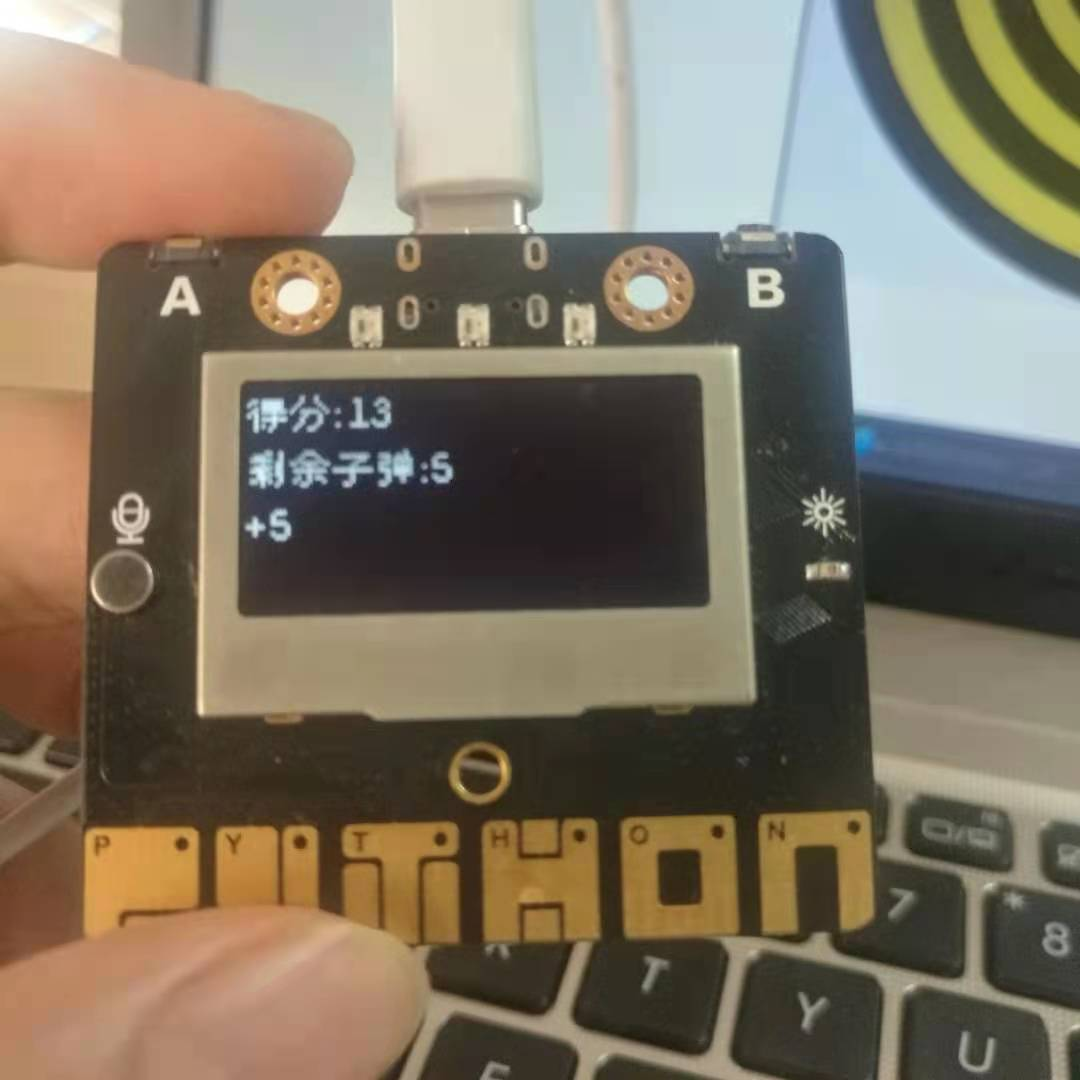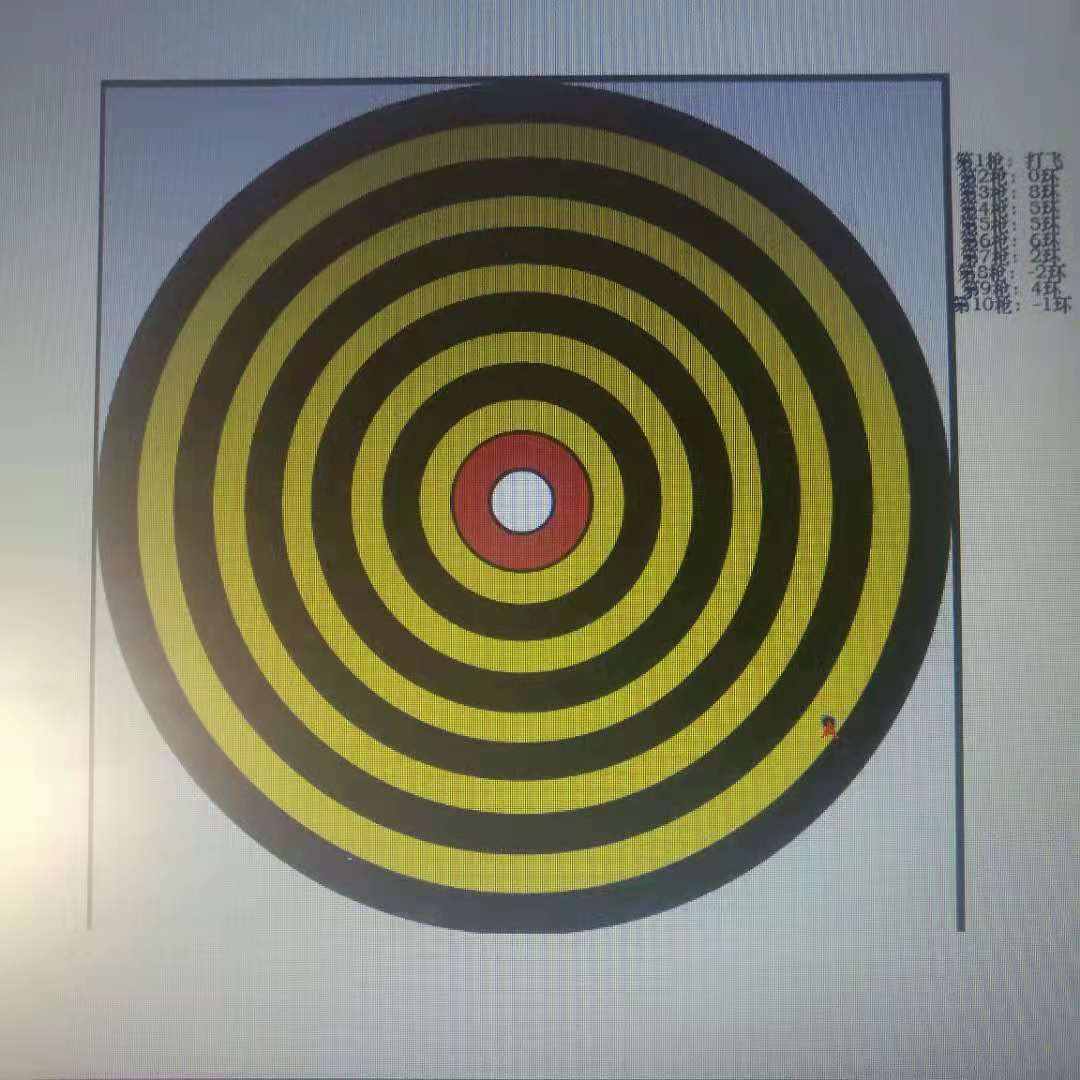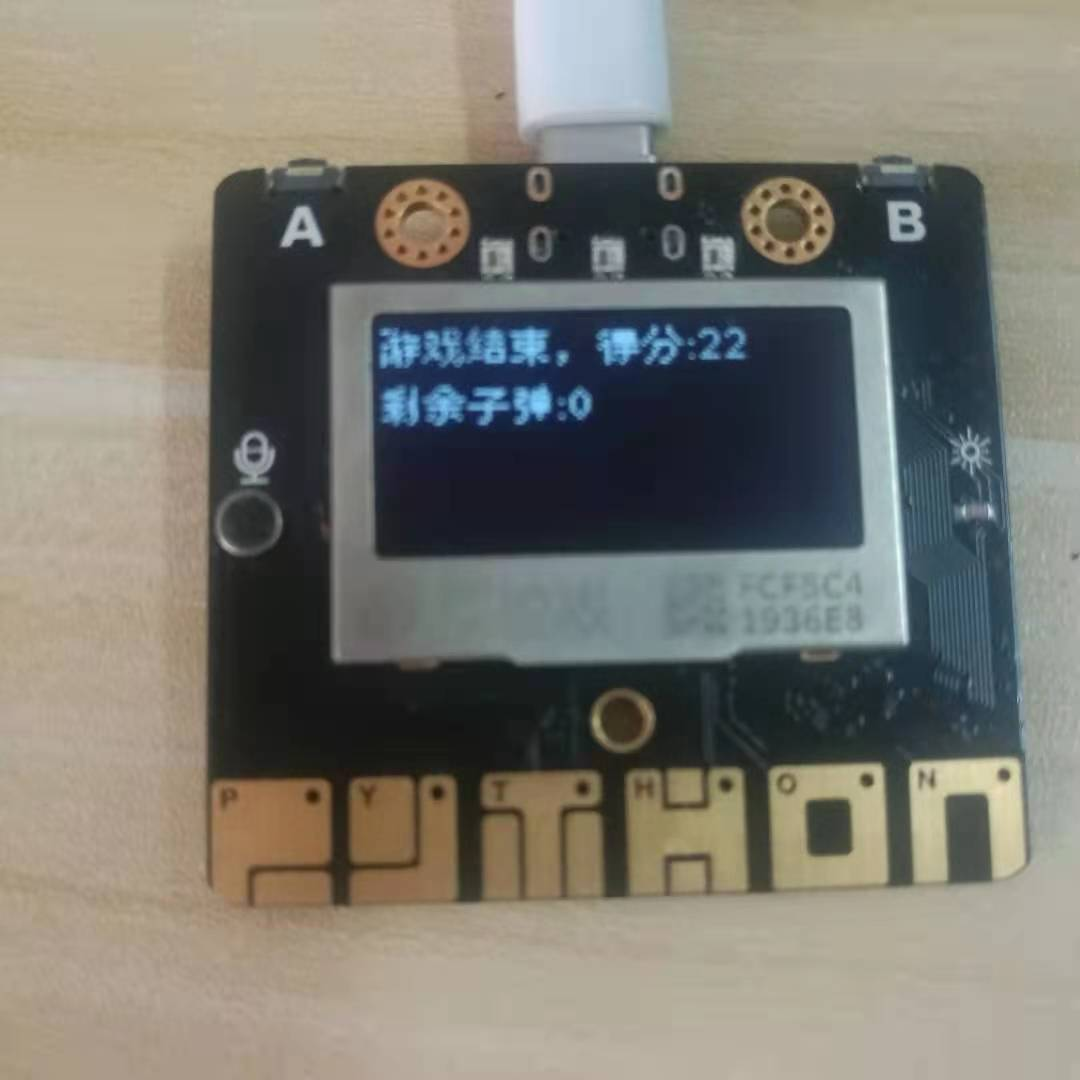Makelog作者原创文章，未经授权禁止转载。
2
0[[c.user_name]] [[c.create_time]]
[[c.parent_comment.count]]
|
[[c.comment_content]]
[[r.user.user_name]] [[r.create_time]]
[[r.comment_content]]

3
11
1
3
5
20
0
7
2
5